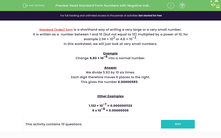# Read Standard Index Form Numbers with Negative Indices

In this worksheet, students will read and write standard form numbers with negative indices as normal numbers.Key stage:  KS 3

Curriculum topic:   Number

Curriculum subtopic:   Interpret/Compare Standard Form Numbers

Difficulty level:#### Worksheet Overview

Standard index form is a shorthand way of writing a very large or a very small number.

It is written as a  number between 1 and 10 (but not equal to 10) multiplied by a power of 10, for example 2.94 × 102 or 4.8 × 10-3.

You can probably see how much easier it is to manage numbers when they are in standard form - counting all those zeros can be quite tricky! It can be incredibly useful in science if you want to compare the magnitude of things that are very large (such as distances in space) or very small (such as the size of bacteria or cells in the body).In this activity, we will just look at very small numbers.

Example

Change 5.83 × 10-6 into a normal number.

We divide 5.83 by 10 six times.

Each digit therefore moves 6 places to the right.

This gives the number 0.00000583.

Other Examples

1.122 × 10-7 = 0.0000001122

6 x 10-8 = 0.00000006

Let's have a a go at some questions.

### What is EdPlace?

We're your National Curriculum aligned online education content provider helping each child succeed in English, maths and science from year 1 to GCSE. With an EdPlace account you’ll be able to track and measure progress, helping each child achieve their best. We build confidence and attainment by personalising each child’s learning at a level that suits them.

Get started